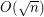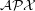# Bentz, Cedric

## Finding k-edge-outerplanar graph embeddings ★★

Author(s): Bentz

Conjecture   It has been shown that a-outerplanar embedding for whichis minimal can be found in polynomial time. Does a similar result hold for-edge-outerplanar graphs?

Keywords: planar graph; polynomial algorithm

## Approximation ratio for k-outerplanar graphs ★★

Author(s): Bentz

Conjecture   Is the approximation ratio for the Maximum Edge Disjoint Paths (MaxEDP) or the Maximum Integer Multiflow problem (MaxIMF) bounded by a constant in-outerplanar graphs or tree-width graphs?

## Approximation Ratio for Maximum Edge Disjoint Paths problem ★★

Author(s): Bentz

Conjecture   Can the approximation ratiobe improved for the Maximum Edge Disjoint Paths problem (MaxEDP) in planar graphs or can an inapproximability result stronger than-hardness?